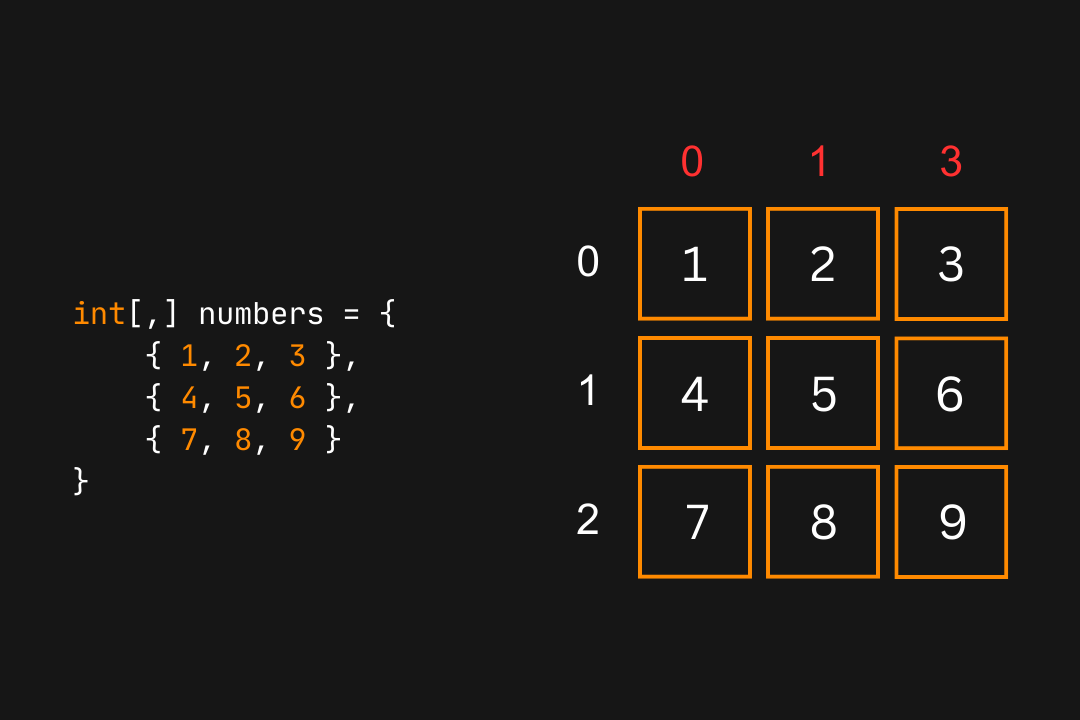Course Content

C# Basics

##Multi-Dimensional Arrays

Arrays can also have additional arrays inside them. Such arrays are called Multi-Dimensional Arrays. These are useful when we want to store the data in a tabular form, with rows and columns, or in the form of a matrix.

We can declare a two-dimensional array using the following syntax:main.csThe array created using the above syntax will have the length (size) equal to `lengthX` and each element will be an array of size `lengthY`. For-example:main.csIn the above case, we create a new two-dimensional matrix of 3x3 size. It means that it can hold `9` integer numbers. We can initialize a 2D array using the following syntax:main.csFor-example:main.csThe above is a 3x4 matrix and it can store 12 elements. The following illustration shows a 3x3 matrix in a visualized form:Indexing in multidimensional arrays is similar to the normal arrays. We simply mention the row and the column index.main.csFor example, if we want to access `6` from the `numbers` array (shown in the illustration), we will access the 2nd row and the 3rd column:main.csHigher dimensional arrays are also possible by adding extra commas to the declaration syntax:main.csIn the above code `myArray3D` will have `60` elements (3x4x5), while ``myArray4D`will have`1800` elements (5x4x9x10).

Following is how you would initialize a 3D array:main.cs